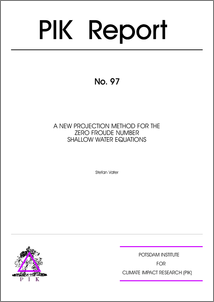Repository: Freie Universität Berlin, Math Department

# A New Projection Method for the Zero Froude Number Shallow Water Equations

Vater, S. (2005) A New Projection Method for the Zero Froude Number Shallow Water Equations. Technical Report. Potsdam Institute for Climate Impact Research.Preview

1MB

## Abstract

For non-zero Froude numbers the shallow water equations are a hyperbolic system of partial differential equations. In the zero Froude number limit, they are of mixed hyperbolic-elliptic type, and the velocity field is subject to a divergence constraint. A new semi-implicit projection method for the zero Froude number shallow water equations is presented. This method enforces the divergence constraint on the velocity field, in two steps. First, the numerical fluxes of an auxiliary hyperbolic system are computed with a standard second order method. Then, these fluxes are corrected by solving two Poisson-type equations. These corrections guarantee that the new velocity field satisfies a discrete form of the above-mentioned divergence constraint. The main feature of the new method is a unified discretization of the two Poisson-type equations, which rests on a Petrov-Galerkin finite element formulation with piecewise bilinear ansatz functions for the unknown variable. This discretization naturally leads to piecewise linear ansatz functions for the momentum components. The projection method is derived from a semi-implicit finite volume method for the zero Mach number Euler equations, which uses standard discretizations for the solution of the Poisson-type equations. The new scheme can be formulated as an approximate as well as an exact projection method. In the former case, the divergence constraint is not exactly satisfied. The &quot;approximateness&quot; of the method can be estimated with an asymptotic upper bound of the velocity divergence at the new time level, which is consistent with the method's second-order accuracy. In the exact projection method, the piecewise linear components of the momentum are employed for the computation of the numerical fluxes of the auxiliary system at the new time level. In order to show the stability of the new projection step, a primal-dual mixed finite element formulation is derived, which is equivalent to the Poisson-type equations of the new scheme. Using the abstract theory of Nicola"ides (1982) for generalized saddle point problems, existence and uniqueness of the continuous problem are proven. Furthermore, preliminary results regarding the stability of the discrete method are presented. The numerical results obtained with the new exact method show significant accuracy improvements over the version that uses standard discretizations for the solution of the Poisson-type equations. In the L-two as well as the L-infinity norm, the global error is about four times smaller for smooth solutions. Simulating the advection of a vortex with discontinuous vorticity field, the new method yields a more accurate position of the center of the vortex.

Item Type: Monograph (Technical Report) Finite Volumes, Projection Methods, Shallow Water Equations Mathematical and Computer Sciences > Mathematics > Numerical Analysis Department of Mathematics and Computer Science > Institute of Mathematics > Geophysical Fluid Dynamics Group 536 Ulrike Eickers 13 Jul 2009 13:07 03 Mar 2017 14:40

Repository Staff Only: item control page